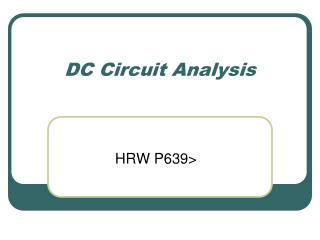Download PresentationDC Circuit Analysis

DC Circuit Analysis - PowerPoint PPT Presentation

DC Circuit Analysis. HRW P639>. Circuit Symbols. Resistor Voltage source (battery) Switch Wire (Conductor). What is a circuit?. A basic circuit comprises: Voltage source (ex. battery) Load (ex. Resistors) Conductor (ex. Wires) Control (ex. Switch). DC Circuit Analysis.I am the owner, or an agent authorized to act on behalf of the owner, of the copyrighted work described.
Download PresentationDC Circuit Analysis

Download Policy: Content on the Website is provided to you AS IS for your information and personal use and may not be sold / licensed / shared on other websites without getting consent from its author.While downloading, if for some reason you are not able to download a presentation, the publisher may have deleted the file from their server.

- - - - - - - - - - - - - - - - - - - - - - - - - - E N D - - - - - - - - - - - - - - - - - - - - - - - - - -
Presentation Transcript
1. DC Circuit Analysis HRW P639>

2. Circuit Symbols • Resistor • Voltage source (battery) • Switch • Wire (Conductor)

3. What is a circuit? • A basic circuit comprises: • Voltage source (ex. battery) • Load (ex. Resistors) • Conductor (ex. Wires) • Control (ex. Switch)

4. DC Circuit Analysis • DC Circuits are analyzed in 3 models • Series resistors • Parallel resistors • Combination resistors • Circuit Analysis investigates: • Equivalent resistance • Circuit current in/out battery • Voltage drop across components • Currentthrough components

5. DC Circuit Analysis - Series • Components are connected “nose-tail” or inline • There is only one path for the circuit current (I) to flow.

6. Examples of series circuits

7. DC Circuit Analysis – Series • Equivalent (or total) resistance • RT = R1 + R2 + R3 • Current is the same through all components = circuit current (I) • Voltage drop across each resistor (n) • Vn = I*Rn • Sum of voltage drops = battery voltage

8. DC Circuit Analysis – Series Practice • Analyze the series circuit by calculating: • Equivalent resistance RT • Circuit current (I) flowing into/out of the battery • Voltage drop across the 3, 4 and 5 ohm resistor

9. DC Circuit Analysis - Parallel • Components are connected “side by side” • There are multiple paths for the current to flow, splitting into branch currents through R1, R2 and R3, according to their resistive values. • The circuit current (I) flows into/out of the battery.

10. Examples of parallel circuits

11. DC Circuit Analysis – Parallel • Equivalent resistance • 1/RT = 1/R1 + 1/R2 + 1/R3 • Voltage is the same across all n resistors (V) • Current in each resistor (In) • In = V/Rn

12. DC Circuit Analysis – Parallel Practice • Analyze a parallel circuit by finding: • Equivalent resistance (RT) • Circuit current (I) • Current (In) through each of the resistors Rn • I1 through R1 • I2 through R2 • I3 through R3

13. DC Circuit Analysis – Combo • Resistors are connected in both series (R3) and parallel (R1, R2) methods • Analyze by starting to simplify from the inside-out: • Simplify R1 and R2 > R12 • Add result (R12) to R3 for total circuit resistance (Rtot) • Calculate circuit current • I = V/Rtot • Calculate currents through selected resistances

14. Parallel part of combo • Consider current (I) • Passes through R3 in series with battery • Splits so that larger part goes through the low value resistor (Rlow) , and smaller part goes through high value (Rhigh) resistor

15. DC Circuit Analysis – Combo Practice • Given V = 12V, R1, R2 and R3 = 1, 2, 3 ohm respectively, calculate: • Equiv resistance (RT) • Circuit current (I) • Current through R2 (I2) • See next slide

16. Combo Shortcut for current • R1 and R2 are in parallel, so the voltage drop across each resistor is the same. • Shortcut for calculating In when there are 2 resistors (Rlow and Rhigh) in parallel: • Ilow = (Rhigh/(Rlow + Rhigh) * circuit current (I) • Ihigh = (Rlow/(Rlow + Rhigh) * circuit current (I) • We are simply apportioning the current according to the resistance • Higher resistance = lower current (and v.v) Ilow = current through Rlow & Ihigh = current through Rhigh